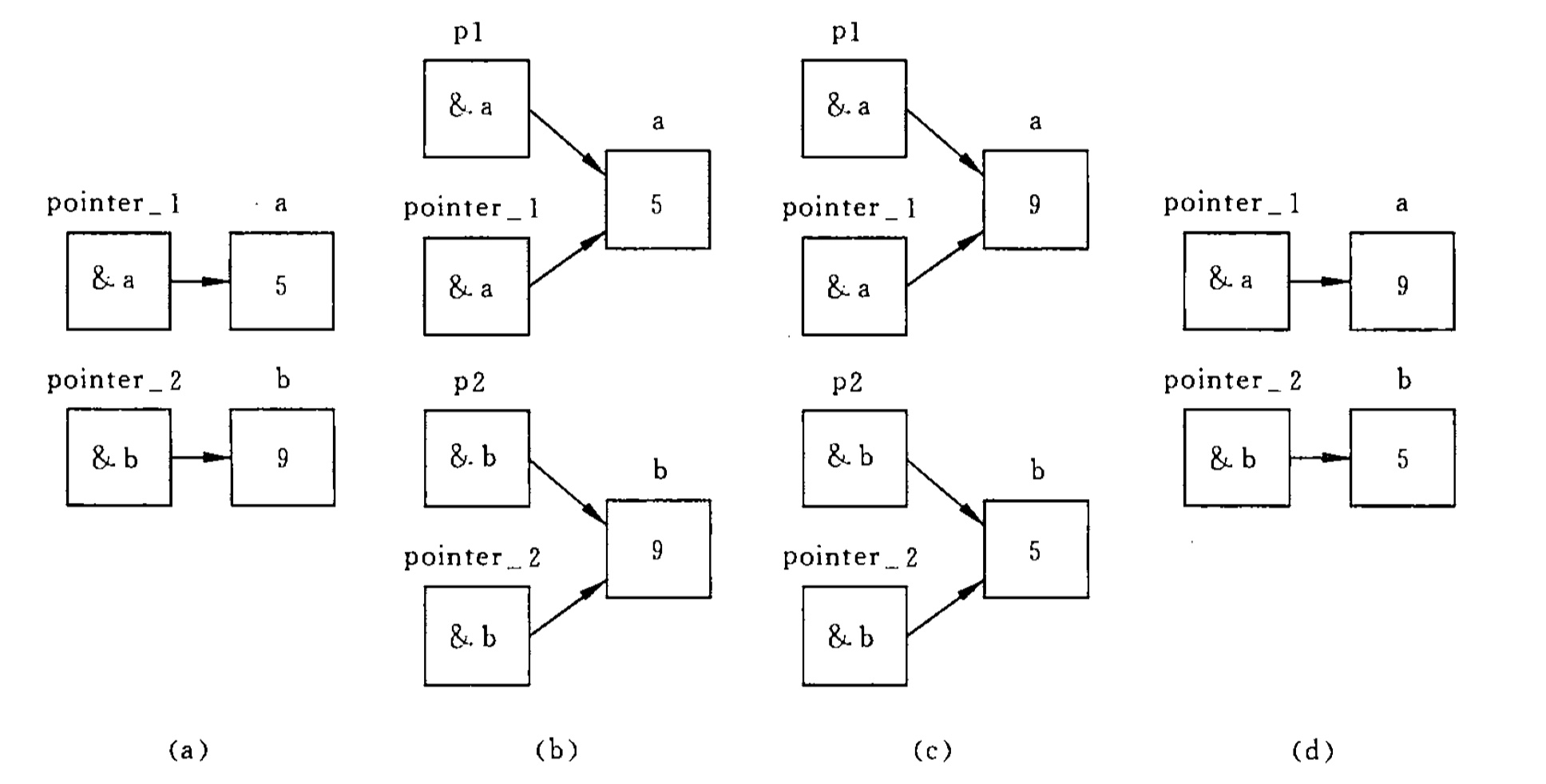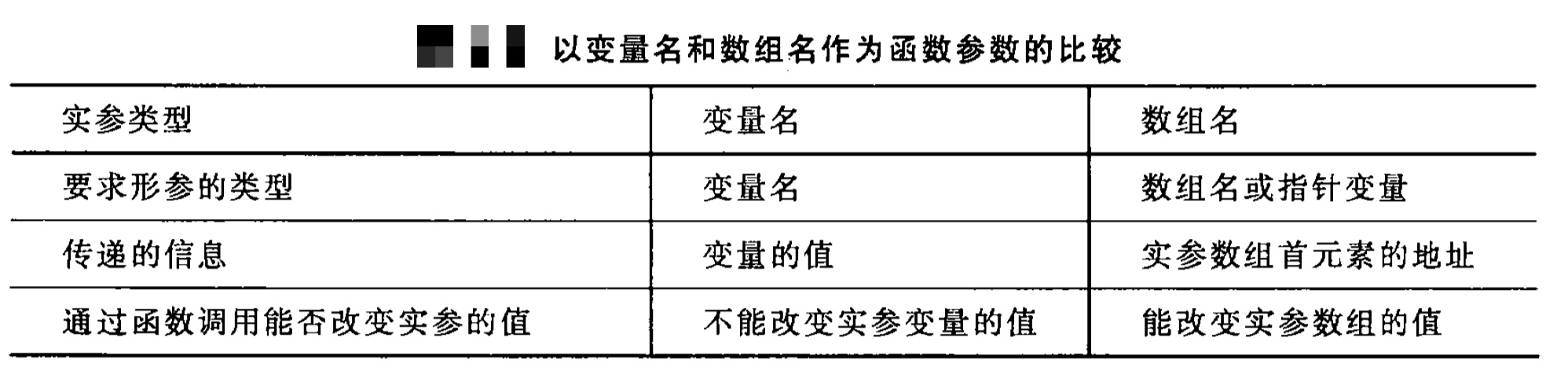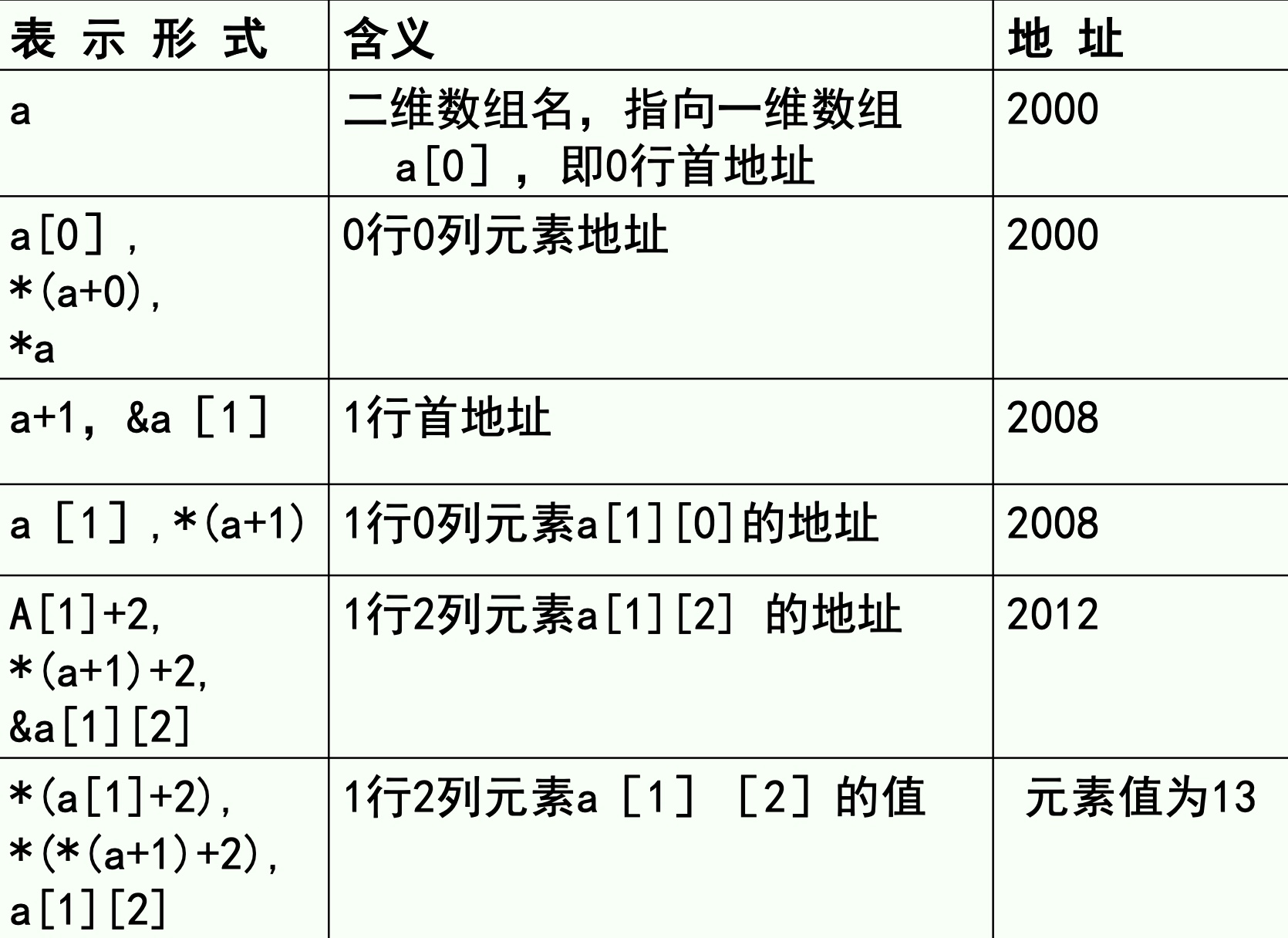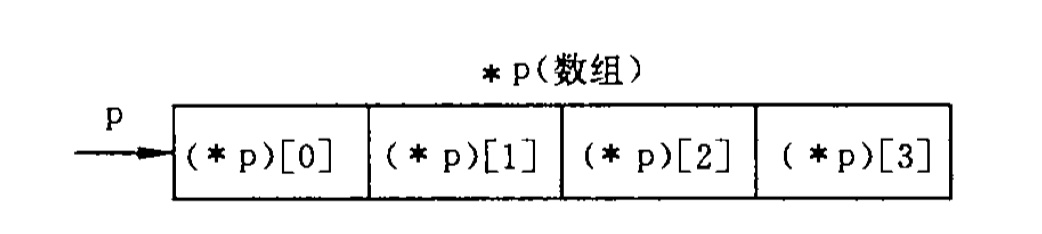# C 语言（考纲总结）：七、指针

10.021k 字  |  34 分钟

## 7.1 地址与指针

• 地址：内存区的每一个字节有一个编号，这就是 地址 。如果在程序中定义了一个变量，在对程序进行编译时，系统就会给这个变量分配内存单元。

• 存取变量值的方式有两种：

• 按变量地址存取变量值的方式称为 直接访问 方式。
• 另一种存取变量值的方式称为 间接访问 的方式。即，将变量 i 的地址存放在另一个变量中。
• 指针：一个变量的地址称为该变量的 指针。由于通过地址能找到所需的变量单元，可以说，地址指向该变量单元。通常我们将地址形象化地称为 「指针」。意思是通过它能找到以它为地址的内存单元。

• 指针变量：如果有一个变量专门用来存放另一变量的地址（即指针），则它称为 指针变量

## 7.2 指针变量：定义和赋值、访问、参数和返回值

### 7.2.1 指针变量的定义和赋值

• 形式：基类型 *指针变量名; 例如：float *pointer_3; char *pointer_4;
• 赋值：使一个指针变量得到另一个变量的地址，从而使它指向一个该变量。例如：pointer_1 = &i; pointer_2 = &j;

1. 指针变量前面的 *，表示该变量的类型为指针型变量。例：float *pointer_1;指针变量名是pointer_1，而不是 * pointer_1

2. 在定义指针变量时必须指定基类型。需要特别注意的是，只有整型变量的地址才能放到指向整型变量的指针变量中。下面的赋值是错误的：float a; int *pointer_1; pointer_1 = &a;

3. 一个变量的指针的含义包括两个方面，一是以存储单元编号表示的地址（如编号为2000的字节），一是它指向的存储单元的数据类型（如 int，char，float 等）。

4. 指向整型数据的指针类型表示为 int *，读作 "指向 int 的指针" 或简称 "int 指针"。可以有 int *, char *, float * 等指针类型，如上面定义的指针变量 pointer_3 的类型是 float *pointer_4 的类型是 char *(int* ), (float* ), (char* )是 3 种不同的类型，不能混淆。

5. 指针变量中只能存放地址(指针),不要将一个整数赋给一个指针变量。如:

*pointer_1 = 100; // pointer_1 是指针变量，100 是整数，不合法
原意是想将地址 100 赋给指针变量 pointer_1，但是系统无法辨别它是地址，从形式上看 100 是整常数，而整常数只能赋给整型变量，而不能赋给指针变量，判为非法。

### 7.2.2 指针变量的引用

1. 给指针变量赋值。如：p = &a; // 把 a 的地址赋给指针变量 p

1. 引用指针变量指向的变量。如果已执行 p = &a;，即指针变量 p 指向了整型变量 a。则
printf("%d", *p); 的作用是以整数形式输出指针变量 p 所指向的变量的值，即变量 a 的值。如果有以下赋值语句：*p = 1; 表示将整数 1 赋给 p 当前所指向的变量，如果 p 指向变量 a，则相当于把 1 赋给 a，即 a = 1;
2. 引用指针变量的值。如：printf("%o", p); 作用是以八进制数形式输出指针变量 p 的值，如果 p 指向了 a，就是输出了 a 的地址，即 &a。
• &* 运算符：
1. & 取地址运算符。&a 是变量 a 的地址。
2. * 指针运算符（或称 "间接访问" 运算符），*p 代表指针变量 p 指向的对象。

1. 指针变量作为函数参数：
# include <stdio. h>
int main()
{
void swap(int x p1,int x p2);                 // 対 swap 函数的声明
int a,b;
int *pointer_1, *pointer_2;                     // 定义两个 int* 型的指针变量
scanf("%d, %d”,&a,&b);                                 // 输入两个整数
pointer_1 = &a;                                             // 使 pointer_1 指向 a
pointer_2 = &b;                                             // 使 pointer_2 指向 b
if (a < b) {                                                 // 如果 a < b, 凋用 swap 函数
swap(pointer_1, pointer_2);
}
printf("max = %d, min = %d\n" ,a,b);  // 输出结果
return 0;
}
void swap(int *p1, int *p2) {                       // 定义 swap 函数
int temp;
temp = *pl;                                                     // 使 *p1 和 *p2 互换
*pl = *p2;
*p2 = temp;
}
• 程序分析：

swap 是用户自定义函数，它的作用是交换两个变量（a 和 b）的值。swap 函数的两个形参 p1 和 p2 是指针变量。程序运行时，先执行 main 函数，输入 a 和b的值（现输入 5 和 9)。然后将 a 和 b 的地址分别赋给 int * 变量 pointer_1 和 pointer_2，使 pointer_1 指向 a，pointer_2 指向 b，见图（a）。接着执行 if 语句，由于 a < b，因此执行 swap 函数。
注意实参 pointer_1 和 pointer_2 是指针变量，在函数调用时，将实参变量的值传送给形参变量，采取的依然是「值传递」方式。因此虚实结合后形参 p1 的值为 &a，p2的值为 &b，见图（b）。这时 p1 和 pointer_1 都指向变量a，p2 和 pointer_2 都指向 b。接着执行 swap 函数的函数体，使 *p1 和 *p2 的值互换，也就是使 a 和 b 的值互换。互换后的情况见图（c）。函数调用结束后，形参 p1 和 p2 不复存在（已释放），情况如图（d）所示。最后在 main 函数中输出的 a 和 b 的值已是经过交换的值（a = 9，b = 5）。## 7.3 指针运算：指针与整数的加减、指针相减和比较、强制类型转换和 void* 指针、不合法的指针运算、指针类型与数组类型的差异

1. 加一个整数（用 + 或 +=），如 p+1；
2. 减一个整数（用 - 或 -=），如 p-1；
3. 自加运算，如 p++，++p；
4. 自减运算，如 p--，--p；
5. 两个指针相减，如 p1-p2（只有 p1 和 p2 都指向同一数组中的元素吋オ有意义）。

1. 如果指针变量p已指向数组中的一个元素，则 p+1 指向同一数组中的下一个元素，p-1 指向同一数组中的上一个元素。注意：执行 p+1 时并不是将 p 的值（地址）简单地加 1，而是加上一个数组元素所占用的字节数。例如，数组元素是 float 型，每个元素占 4 个字节，则 p+1 意味着使 p 的值（地址）加 4 个字节，以使它指向下一元素。p+1 所代表的地址实际上是 (p+1)*d，d 是一个数组元素所占的字节数（ Visual C++ 6.0 中，对 int 型，d = 4；对 float 和 long 型，d = 4；对 char 型，d = 1）。若 p 的值 2000，则 p+1 的值不是 2001，而是 2004。

系统怎么知道要把这个 1 转换为 4，然后与 p的值相加呢？

在定义指针变量时要指定基类型，如：float *p; // 指针变量 p 的基类型为 float
现在 p 指向 float 型的数组元素，在执行 ++p 时，系统会根据 p 的基类型为 float 型而将其值加 4，这样，p 就指向 float 型数组的下一个元素。如果 p 原来指向 a，执行 ++p 后 p 的值改变了，在 p 的原值基础上加 d，这样 p 就指向数组的下一个元素 a

2. 如果 p 的初值为 &a，则 p+ia+i 就是数组元素 a[i] 的地址，或者说，它们指向 a 数组序号为 i 的元素。这里需要注意的是 a 代表数组首元素的地址，a+1 也是地址，它的计算方法同 p+1，即它的实际地址为 (a+1)*d。例如，p+9a+9 的值是 &a，它指向 a

3. *(p+i)*(a+i)p+ia+i 所指向的数组元素，即 a[i]。例如，*(p+5)*(a+5) 就是a。即 *(p+5)*(a+5)a 三者等价。实际上，在编译时，对数组元素 a[i] 就是按 *(a+i) 处理的，即按数组首元素的地址加上相对位移量得到要找的元素的地址,然后找出该单元中的内容。若数组 a 的首元素的地址为 1000，设数组为 float 型，则 a 的地址是这样计算的： 1000+3*4=1012，然后从 1012 地址所指向的 float 型单元取出元素的值，即 a 的值。可以看出，[] 实际上是变址运算符，即将 a[i]a+i 计算地址，然后找出此地址单元中的值。

4. 如果指针变量 p1p2 都指向同一数组，如执行 p2-p1，结果是 p2-p1 的值（两个地址之差）除以数组元素的长度。假设 p2 指向实型数组元素 ap2 的值为 2020p1 指向 a，其值为 2012，则 p2-p1 的结果是 (2020-2012)/4=2。这个结果是有意义的，表示 p2 所指的元素与 p1 所指的元素之间差 2 个元素。这样，人们就不需要具体地知道 p1p2的值，然后去计算它们的相对位置，而是直接用 p2-p1 就可知道它们所指元素的相对距离。两个地址不能相加，如 p1+p2 是无实际意义的。

### 7.3.1 指针变量加（减）一个整数

p++p--p+ip-ip += ip -= i 等。

### 7.3.2 指针变量赋值

1. p = &a; （将变量 a 的地址赋给 p）

2. p = array;（将数组 array 首元素地址赋给 p）

3. p = &array[i];（将数组 array 第 i 个元素的地址赋给 p）

4. p = max;（max 为已定义的函数，将 max 的入口地址赋给 p）

5. p1 = p2;（p1 和 p2 都是指针变量，将 p2 的值赋给 p1）

### 7.3.6 void 指针类型

• void 指针类型：即可定义一个指针变量，但不指定它是指向哪一种类型数据的。ANSIC 标准规定用动态存储分配函数时返回 void 指针，它可以用来指向一个抽象的类型的数据，在将它的值赋给另一指针变量时要进行强制类型转换使之适合于被赋值的变量的类型。例如：
char []*p1;
void []*p2;
……
p1 = (char *)p2;
• 同样可以用 (void *)p1p1 的值转换成 void *类型。如：p2 = (void *)p1;

• 也可以将一个函数定义为void * 类型，如：void *fun(char ch1, char ch2)表示函数 fun 返回的是一个地址，它指向 "空类型"，如需要引用此地址，也需要根据情况对之进行类型转换，如对该函数调用得到的地址要进行以下转换：p1 = (char *)fun(ch1, ch2);

## 7.4 指针与数组

• 数组元素的指针：

• 指向数组元素的指针变量定义方法：
int a = {1,3,5,7,9,11,13,15,17,19}; //定义a为包含10个整型数据的数组
int * p; // 定义 p 为指向整型变量的指针变量
p = &a;  // 把a 元素的地址赋给指针变量 p
• 对指向数组元素的指针变量赋值：p = &a;

• a 元素的地址赋给指针变量 p。也就是使 p 指向 a 数组的第 0 号元素。

• 通过指针引用数组元素：

1. 下标法，如 a[i] 形式
2. 指针法，如 *(a+i)*(p+i)
• 其中 a 是数组名，p 是指向数组元素的指针变量，其初值 p == a
• 用数组名作函数参数：

• 实参用数组名，也可用指针变量名；形参可用数组名，也可用指针变量名。
• 实参数组名代表该数组首元素的地址，而形参是用来接收从实参传递过来的数组首元素地址的。因此，形参应该是一个指针变量（只有指针变量才能存放地址）。实际上，C 编译都是将形参数组名作为指针变量来处理的。
void f(int arr[], int n) {} /* 形参 arr 为数组名 */
// 等价于：
void f(int *arr, int n) {} /* 形参 arr 为指针变量 */C 语言调用函数时虚实结合的方法都是采用「值传递」方式，当用变量名作为函数参数时传递的是变量的值，当用数组名作为函数参数时，由于数组名代表的是数组首元素地址，因此传递的值是地址，所以要求形参为指针变量。

## 7.5 指向二维数组的指针、多重指针和指针数组

### 7.5.1 多维数组元素的地址### 7.5.2 指向多维数组元素的指针变量

1. 指向数组元素的指针变量
// 有一个 3*4 的二维数组，要求用指向元素的指针变量输出二维数组各元素的值。
#include <stdio.h>
int main()
{
int a = {1,3,5,7,9,11,13,15,17,19,21,23};
int *p;                                                                 // p 是 int * 型指针变量
for(p = a; p < a+12; p++) {            // 使 p 依次指向下一个元素
if((p-a)%4 == 0) {                                   // p 移幼 4 次后换行
printf("\n”);
}
printf(" %4d", *p);                                       // 输出 p 指向的元素的值
}
printf("\n");
}
1. 指向由 m 个元素组成的一维数组的指针变量
#include <stdio.h>
int main()
{
int a = {1,3,5,7,9,11,13,15,17,19,21,23}; // 定义二维数组 a 并初始化
int (*p),i,j;                                                                // 指针变量 p 指向包含 4 个整型元素的一维数组
p = a;                                                                                  // p 指向二维数组的 0 行
scanf("%d, %d" , &i, &j);                                             // 输入要求输出的元素的行列号
printf("a[%d,%d] = %d\n",i,j,*(*(p+i)+j));            // 输出 a[i][j] 的值
return 0;
}

(* p)p二种形式比较：

1. int a; （a 有 4 个元素，每个元素为整型）
2. int (*p);表示 (*p) 有 4 个元素，每个元素为整型。也就是 p 所指的对象是有 4 个整型元素的数组，即 p 是指向一维数组的指针，见图。应该记住，此时 p 只能指向一个包含 4 个元素的一维数组，不能指向一维数组中的某一元素。p 的值就是该一维数组的起始地址。1. 用指向数组的指针作函数参数

1. 用指向变量的指针变量；
2. 用指向一维数组的指针变量。
// 有一个班，3 个学生，各学 4 门课，计算总平均分数以及第 n 个学生的成绩。
#include <stdio.h>
int main()
{
void average(float *p, int n);
void search(float (*p), int n);
float score = { {165,67,70,60}, {80,87 ,90,81}, {90,99,100,98} };
average(*score, 12);        // 求 12 个分数的平均分
search(score, 2);               // 求序号为 2 的学生的成绩
return 0;

}

void average(float *p,int n) // 定义求平均成绩的函数
{
float *p_end;
float sum = 0, aver;
p_end = p+n-1;  // n 的值为 12 吋，p_end 的值是 p+11，指向最后一个元素
for( ; p<=p_end; p++) {
sum = sum+(*p);
}
aver= sum/n;
printf('average = %5.2f\n",aver);
}

void search(float (*p),int n) // p 是指向具有 4 个元素的一维数组的指针
{
int i;
printf("The score of No. %d are:\n", n);
for(i = 0; i < 4; i++)
printf("%5.2f", *(*(p+n)+i));
printf("\n");
}

1. 在 main 函数中，先调用 average 函数以求总平均值。在函数 average 中形参 p 被声明为 float* 类型（指向 float 型变量）的指针变量。它的基类型为 float 型，实参用 *score, 即 score，也就是 &score，即 score 的地址。把 score 的地址传给 p，使 p 指向 score。然后在 average 函数中使 p 先后指向二维数组的各个元素，p 每加 1 就改为指向 score 数组的下一个元素。形参 n 代表需要求平均值的元素的个数，实参 12 表示要求 12 个元素值的平均值。p_end 是最后一个元素的地址。sum 是累计总分，aver 是平均值。在函数中输出 aver 的值，函数无需返回值。
2. 函数 search 的形参 p 的类型是 float(*)，它不是指向整型变量的指针变量，而是指向包含 4 个元素的一维数组的指针变量。函数调用开始时，将实参 score 的值（代表该数组 0 行起始地址）传给 p，使 p 也指向 scorep+nscore[n] 的起始地址，*(p+n)+iscore[n][i]的地址，*(*(p+n)+i)score[n][i] 的地址。现在实参传给形参 n 的值是2，即想找序号为 2 的学生的成绩（3 个学生的序号分别为0，1，2）。
3. 调用 search 函数时，实参是 score（二维数组名，代表该数组 0 行起始地址）传给 p，使 p 也指向scorep+nscore[n] 的起始地址，*(p+n)+iscore[n][i] 的地址，*(*(p+n)+i)score[n][i] 的值。现在 n = 2，i 由 0 变到 3，for 循环输出 scorescore 的值。

## 7.6 函数指针

### 7.6.1 用函数指针变量调用函数

#include <stdio.h>

int main()
{
int max(int, int);                        // 函数声明
int (*p)(int,int);                      // 定义指向函数的指针变量 p
int a, b, c;
p = max;                                            // 使 p 指向 max 函数
scanf("%d,%d", &a, &b);
c = (*p)(a,b);                              // 通过指针变量调用 max 函数
printf("a= % d\nb= % d\nmax= %d\n”" ,a,b,c);
return 0;
}

int max(int x,int y)                        // 定义 max 函数
{
int z;
if(x > y) {
z = x;
} else {
z = y;
}
return (z);
}

### 7.6.2 用指向函数的指针作函数参数

// 实参函数名：f1，f2
void fun (int (*x1)(int), int(*x2)(int, int)) // 定义 fun 函数，形参是指向函数的指针变量
{
int a, b, i = 3, j = 5;
a = (*x1)(i);                   // 调用 f1 函数，i 是实参
b = (*x2)(i,j);             // 调用 f2 函数，i，j 是实参
}

### 7.6.3 返回指针值的函数

*a 两侧没有括号，在 a 的两侧分别为 * 运算符和 () 运算符。而 () 优先级高于 *，因此 a 先与 () 结合，显然这是函数形式。这个函数前面有一个 *，表示此函数是指针型函数（函数值是指针）。最前面的 int 表示返回的指针指向整型变量。

// 有 a 个学生，每个学生有 b 门课程的成绩。要求在用户输人学生序号以后，能输出该学生的全部成绩。用指针函数来实现。
#include <stdio.h>
int main()
{
float score[] ={ {60,70,80,90}, {56,89,67,88}, {34,78,90,66} }; // 定义数组，存放成绩
float *search(float (*pointer), int n);  // 函数声明
float *p;
int i, k;
printf("enter the number of student!");
scanf("%d", &k);                                                      // 输入要找的学生的序号
printf("The scores of No.%d are:\n", k);
p = search(score,k);                                                // 调用 search 函数，返回 score[k] 的地址
for(i = 0; i < 4; i++)
printf("%5.2ft", *(p+i));                                 // 输出 score[k]~score[k] 的值
printf("n");
return 0;
}

float *search(float (*pointer), int n)           // 形参 pointer 是指向一维数组的指针变量
{
float * pt;
pt = *(pointer-n);                                                  // pt 的值是 score[k]
return(pt);
}

# 评论（没有评论）# 程序员充电站微信支付支付宝